## 5.2.2 Simulation and Parameter Extraction

For the transient simulation of the temperature evolution the three-dimensional interconnect simulator STAP  has been used within the simulation and optimization framework SIESTA. STAP calculates for isotropic materials JOULE's self-heating effect by solving the continuity equation (2.10) and the heat conduction equation (2.53), which is coupled to the power loss equation (2.54). For these investigations all material parameters are assumed to be isotropic and for the thermal and the electrical conductivity a temperature-dependence is approximated by a polynomial models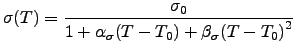(5.5)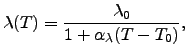(5.6)

where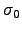and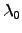are the conductivities at a certain reference temperature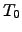and,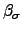, and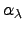are the corresponding first and second order temperature coefficients. which represent the target for the parameter extraction.

For the parameter identification, the initial values for the thermal and electrical conductivity of polySi and polycide were obtained from literature [343,137,344,136,25]. A gradient-based optimization strategy has been used to improve the initial values in order to achieve good agreement with the reference data obtained from the measurements.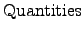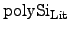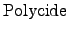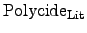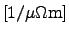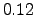-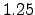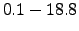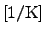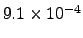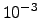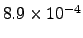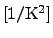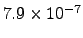-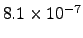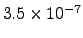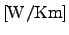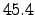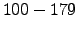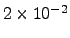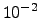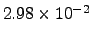-

Stefan Holzer 2007-11-19